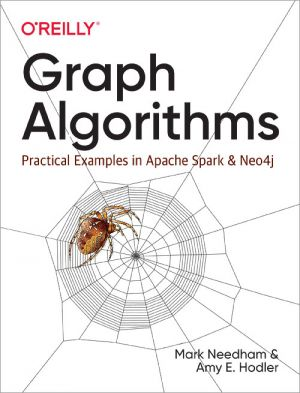# Graph Algorithms

## Practical Examples in Apache Spark and Neo4j

by Mark Needham, Amy HodlerJoin

### Book Description

Learn how graph algorithms can help you leverage relationships within your data to develop intelligent solutions and enhance your machine learning models. With this practical guide, developers and data scientists will discover how graph analytics deliver value, whether they're used for building dynamic network models or forecasting real-world behavior.

Mark Needham and Amy Hodler from Neo4j explain how graph algorithms describe complex structures and reveal difficult-to-find patterns - from finding vulnerabilities and bottlenecks to detecting communities and improving machine learning predictions. You'll walk through hands-on examples that show you how to use graph algorithms in Apache Spark and Neo4j, two of the most common choices for graph analytics.

Learn how graph analytics reveal more predictive elements in today's data; Understand how popular graph algorithms work and how they're applied; Use sample code and tips from more than 20 graph algorithm examples
Learn which algorithms to use for different types of questions; Explore examples with working code and sample datasets for Spark and Neo4j; Create an ML workflow for link prediction by combining Neo4j and Spark

Chapter 1
Introduction
Chapter 2
Graph Theory and Concepts
Chapter 3
Graph Platforms and Processing
Chapter 4
Pathfinding and Graph Search Algorithms
Chapter 5
Centrality Algorithms
Chapter 6
Community Detection Algorithms
Chapter 7
Graph Algorithms in Practice
Chapter 8
Using Graph Algorithms to Enhance Machine Learning
Appendix A
Index

### Book Details

Title
Graph Algorithms
Subject
Computer Science
Publisher
O'Reilly Media
Published
2019
Pages
257
Edition
1
Language
English
ISBN13
9781492047681
ISBN10
1492047686
ISBN13 Digital
9781492057819
ISBN10 Digital
1492057819
PDF Size
10.8 MB### Related BooksThis book is assembled from lectures given by the author over a period of 10 years at the School of Computing of DePaul University. The lectures cover multiple classes, including Analysis and Design of Algorithms, Scientific Computing, Monte Carlo Simulations, and Parallel Algorithms. These lectures teach the core knowledge required by any scientis...Algorithms are the lifeblood of computer science. They are the machines that proofs build and the music that programs play. Their history is as old as mathematics itself. This book is a wide-ranging, idiosyncratic treatise on the design and analysis of algorithms, covering several fundamental techniques, with an emphasis on intuition and the proble...The purpose of this book is to give you a thorough introduction to competitive programming. It is assumed that you already know the basics of programming, but no previous background in competitive programming is needed. The book is especially intended for students who want to learn algorithms and possibly participate in the International Olympi...Computer graphics programming books are often math-heavy and intimidating for newcomers. Not this one. Computer Graphics from Scratch takes a simpler approach by keeping the math to a minimum and focusing on only one aspect of computer graphics, 3D rendering. You'll build two complete, fully functional renderers: a raytracer, which simulate...Applied Combinatorics is an open-source book for a course covering the fundamental enumeration techniques (permutations, combinations, subsets, pigeon hole principle), recursion and mathematical induction, more advanced enumeration techniques (inclusion-exclusion, generating functions, recurrence relations, Polyá theory), discrete structures (grap...This is an introductory book on algorithmic graph theory. Theory and algorithms are illustrated using the Sage open source mathematics software....